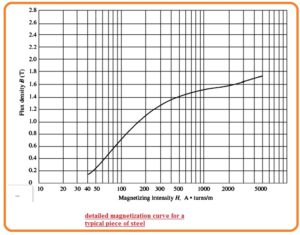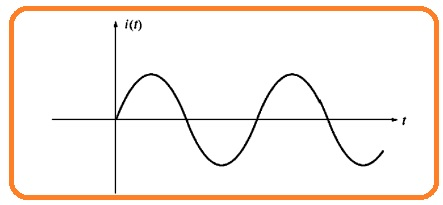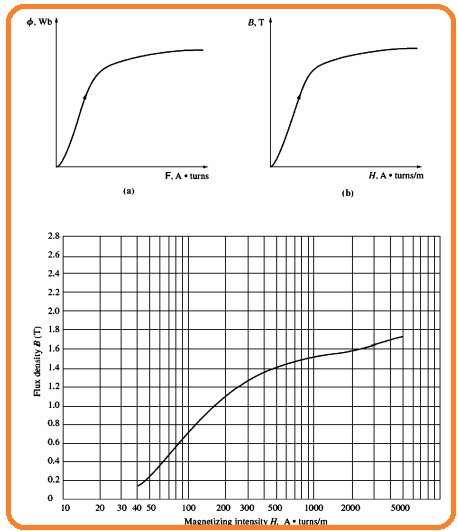Hi, readers welcome to the new tutorial. In this post, we cover the Magnetic Behavior of Ferromagnetic Materials. There are numerous types of magnetism like ferromagnetism is one of them. Such substances show magnetization behavior on its own at atoms in the existence of exterior field. If these substances is put in the magnetic field will get magnetized in the field direction

The ferromagnetic substance shows attraction towards the magnets. If the external field removed about the ferromagnetic substances it still retains the magnetization. This feature is known as hysteresis and to removed the hysteresis there is a need of an exterior field. Here we cover basics details about Ferromagnetic Materials and different parameters. So let get started with  Magnetic Behavior of Ferromagnetic Materials .

## Magnetic Behavior of Ferromagnetic Materials

• The formula for magnetic permeability is

B=μH

• The value of permeability of ferromagnetic material is large almost 600 times the permeability of free space.
• The permeability is taken as constant irrespective of the magnetomotive force given to the substance.
• Though permeability value is constant for free space but it not apply for iron and ferromagnetic substances.
• To define the parameters of magnetic permeability for ferromagnetic substances use dc current at the core as shown in the below figure.• Initial its value is zero amperes and increases to the certain value of current.
• If the flux generated in the core and magnetomotive force is drawn in graph form the results curve is shown here.• This category of the graph is known as the saturation curve or magnetization curve. A start the small increment in magnetomotive force generates a large value current in the consequence flux.
• After a certain location, more increment in the magnetomotive force is generated less increment in flux.
• At last the increment in the magnetomotive force generates zero variation. The area of this diagram where curve is flat is known as saturation region and core is called saturated.
• The place where the flux varies very fastly is known as saturation region of curve and core is called unsaturated.
• The transition region among the unsaturated region and saturated region is known as the knee of the curve.
• Observe that flux generated in the core is linear according to the given mmf in unsaturated region and has constant parameters irrespective to the MMF in saturated region.
• Other curve can seen here.• In this diagram magnetic flux density b is drawn with the magnetizing intensity H.
• According to below-shown equation.• It is can see that magnetizing intensity has a direct relation to the magnetomotive force and flux density is direct to the flux of core.
• So the relation among flux density and magnetic intensity has similar nature among the flux and MMF.
• The slop of curvature of flux density with magnetizing intensity for any value of H is shown in figure• The curve indicates the permeability is high and relatively constant value in unsaturated region and after that reduces to less value like the core is now highly saturated.
• The diagram below is the magnetization curve for normal component of steel indicates the further details and having the magnetizing intensity at log parameters.• The benefit of the use of the ferromagnetic substance for cores in electrical machines like motor and generators and transformer  is which has high value of flux for MMF through iron than having iar.
• If the resultant flux is proportional therefore the given MMF. So core should be work in the unsaturated region of the magnetization graph.

## Energy Losses in Ferromagnetic Core

• In place of giving dc current to the coils of core now provided ac and see results. The current given can be seen here.• Let us suppose that flux in the core at the start is 0. With the current increment at start, the flux in core exists at place ab can seen here.• Generally, saturation curvature can seen here.• though the current decreases the flux exists at different locations through one its moves when current rises.
• With the current decrement, the flux in the core exist at location bcd, and after that when the current rises the flux exist at deb.
• Note that the quality of flux exist in the core relies not for the quantity of current given to coil.
• The reliance at the previous results and resulting faults to re-get location flux location is called hysteresis loop.
• Observe that if high-value MMF is start given to the core and after that diminished.
• The flux path in the core is not 0. In place, a magnetic field exists in the core. This field is known as the residual flux. It is accurately the way permanent magnets is generated.
• To caused the flux to zero the quantity of MMF is called coercive MMF.

### Why hysteresis exist

• To observe the nature of ferromagnetic substances it is compulsory to know the data about the behavior.
• The atoms of iron and like metallic substances such as nickel, cobalt. etc caused to show magnetic fields are in a straight line to one another.
• In metallic substances, there is numerous small size areas known as domains. For every domains all the atoms are in straight lines with fields in a similar direction, therefore, every domain in the substance behaves like a smaller dimensions magnet.
• The cause which complete component of iron looks to has zero flux which many small domains are directed in random direction.
• The instance of the domain structure in the component of iron is can seen here.• If exterior magnetic field is given to the iron it results the domain which directed according to field in results of domains direction in other directions.
• Domains directed in direction of field rises since the atom at end point aligned with the given field.
• The additional atoms get aligned to the field increment the magnetic flux in the iron that in results further increment of straight of field.
• It is a positive feedback factor that results in iron to show permeability larger than the air.
• With the extent of exterior magnetic field rises the total domains which are directed in not accurate direction get directed to the field.
• At last when all atoms and domains in iron are aligned to the exterior field the more increment in MMF can result in a similar flux increment which it will in free space.
• At this location, the iron is get saturated to the flux. It is the condition for the saturated region of the magnetization curve in below figure.• The factor to hysterics is when exterior field is eliminated the domains does not totally get random condition again.
• The cause that domain remains to align is that to move atoms there is a need of energy.
• Generally, the energy given through the exterior field to make the aligned. IF the field is eliminated so there is zero energy supply to results all the domain to get the unaligned state. This type of iron known as a permanent magnet.

That is detailed post about Magnetic Behavior of Ferromagnetic Materials  if still you have any query ask in comments. Thanks for reading have a nice day.